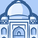# QlikView App Dev

Discussion Board for collaboration related to QlikView App Development.

Announcements
Customer & Partners, DEC. 9, 11 AM ET: Qlik Product & Strategy Roadmap Session: Data Analytics REGISTER NOW
cancel
Showing results for
Did you mean:Creator II

## Formatting issue in a nested if

Hello

I have written the following expression, which when used within a pivot table, the formatting works perfectly

If(v_Selected=1, Num(Count({\$<FactDateNum = {">=\$(=v_Start_Date_IP)<=\$(=v_End_Date_IP)"}>}IP_Spell_No),'#,###'),

If(v_Selected=2, Num(avg({\$<FactDateNum = {">=\$(=v_Start_Date_IP)<=\$(=v_End_Date_IP)"}>}IP_Spell_LOS), '#,##0.00'),

If(v_Selected=3, Num(avg({\$<FactDateNum = {">=\$(=v_Start_Date_IP)<=\$(=v_End_Date_IP)"}>}IP_PreOP_LOS), '#,##0.00') ,

If(v_Selected=4, Num(avg({\$<FactDateNum = {">=\$(=v_Start_Date_IP)<=\$(=v_End_Date_IP)"}>}IP_PostOP_Los),'#,##0.00')

))))

When I store the expression in my expression spreadsheet and load it into my QVW to use as a variable, the formatting does not seem to work anymore

\$(v_IP_Ave_LOS_Count_Spells_Nested_If)

Can anyone help me with why the expression does not work when stored and used as a variable

Kind Regards

Helen

7 RepliesMVP

Do you see a '=' sign next to the variable definition in variable overview? You might need that if that is missing I thinkPartner

Does the expression not work at all, or is it just not applying the formats correctly? If the latter, what results are you getting and what do you expect to get?

Logic will get you from a to b. Imagination will take you everywhere. - A EinsteinCreator II
Author

Hello Jonathan

Thank you for your response.  When using the variable, I am getting values such as:

\$3e+005\$\$\$ but when I use the expression on its own I am getting the desired values I.e. 2,345

Do you think formatting may be the issue?

Kind Regards

HelenPartner

The \$ signs are a little peculiar, but it seems that the formatting is 'lost' when the variable expands, although I can't say why. I suggest that you experiment with the format settings on the Number tab of the chart/table properties. Do you have "expression default" checked for this expression?

Logic will get you from a to b. Imagination will take you everywhere. - A EinsteinPartner

You might also want to try the expression in a new straight/pivot table with the same dimensions, just to make sure that it is not corruption of the expression in the existing table.

Logic will get you from a to b. Imagination will take you everywhere. - A EinsteinCreator II
Author

Hi There

I have tried the expression in a new pivot table and get the same problem

The pivot table is set to Expression Default when the problem occurs, but if I change it to an integer or Fixed decimals, I get values again.   I would like to just change the formatting on the number tab, but as 1 of the if statements requires a different formatting from the others, I can not set them all to the same format

Thank you for your suggestions.  For now, I have referred back to my original expression and am not using the variable at the present time

Kind Regards

HelenSpecialist III

Hi helen_pip,

=Pick(Selected,

Num(Count({\$<FactDateNum = {">=\$(=v_Start_Date_IP)<=\$(=v_End_Date_IP)"}>}IP_Spell_No),'#,##0','.',','),

Num(avg({\$<FactDateNum = {">=\$(=v_Start_Date_IP)<=\$(=v_End_Date_IP)"}>}IP_Spell_LOS), '#,##0.00','.',','),

Num(avg({\$<FactDateNum = {">=\$(=v_Start_Date_IP)<=\$(=v_End_Date_IP)"}>}IP_PreOP_LOS), '#,##0.00','.',','),

Num(avg({\$<FactDateNum = {">=\$(=v_Start_Date_IP)<=\$(=v_End_Date_IP)"}>}IP_PostOP_Los),'#,##0.00','.',',')

)

RegardsCommunity Browser